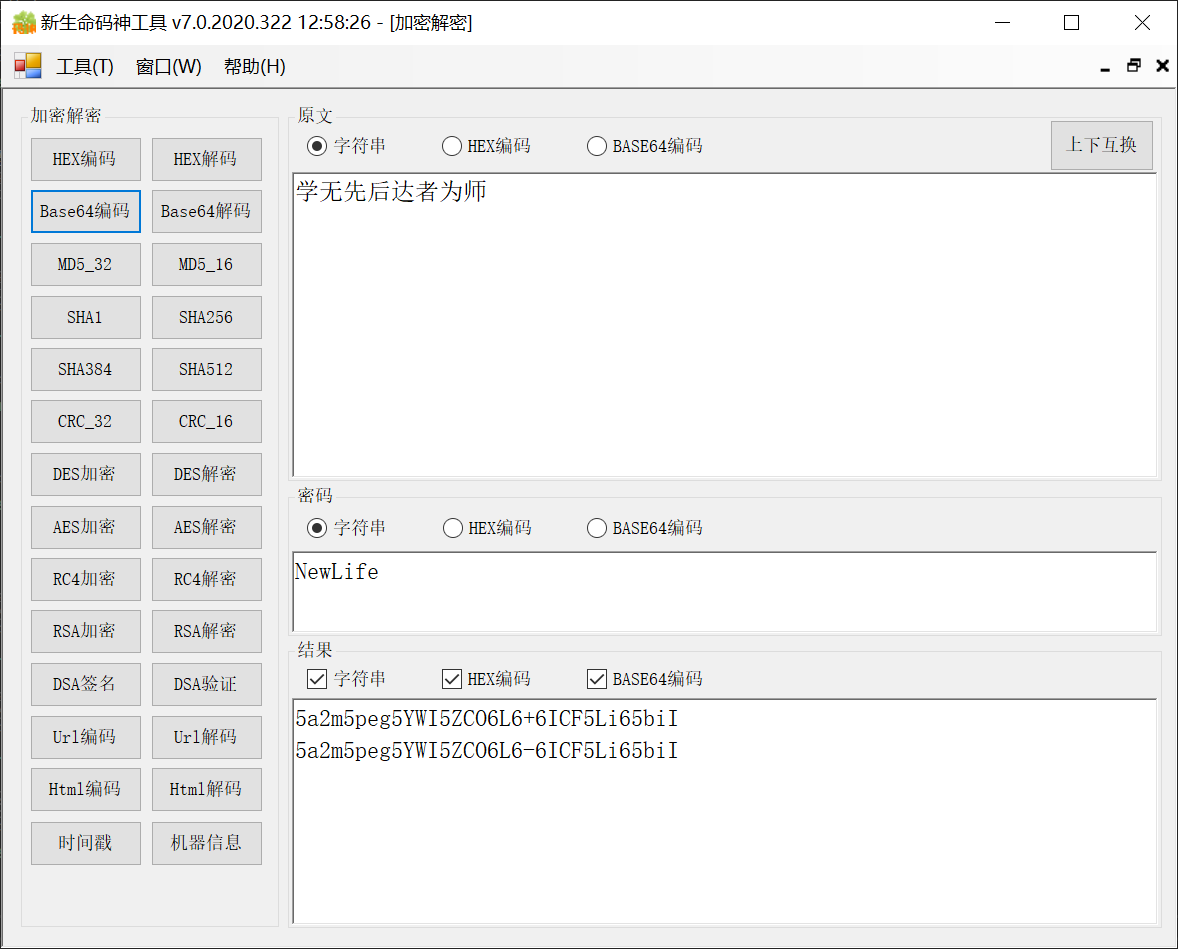IOHelper主要是针对数据流Stream和字节数组Byte[]的扩展。

X组件在协议（包括通信协议和文件协议）上有极为深厚的积累和支持，完全依托于该扩展。各种协议包括但不限于：Http/Redis/Zip/SRMP/RocketMQ/MySql/Thrift/Hive

Nuget包：NewLife.Core

# 压缩与解压缩

Compress/Decompress 支持对数据流和字节数据进行压缩和解压缩，内置Deflate压缩算法；

CompressGZip/DecompressGZip 则是GZip压缩算法，适用于http压缩，或者单文件压缩；

# 数据流读写

`Byte[] ReadBytes(this Stream stream, Int64 length = -1)`

## ToStr

```String ToStr(this Stream stream, Encoding encoding = null);
String ToStr(this Byte[] buf, Encoding encoding = null, Int32 offset = 0, Int32 count = -1);```

```Stream WriteArray(this Stream des, params Byte[] src);

```Stream WriteDateTime(this Stream stream, DateTime dt);

# 字节数组与整数互转

```UInt16 ToUInt16(this Byte[] data, Int32 offset = 0, Boolean isLittleEndian = true);
UInt32 ToUInt32(this Byte[] data, Int32 offset = 0, Boolean isLittleEndian = true);
UInt64 ToUInt64(this Byte[] data, Int32 offset = 0, Boolean isLittleEndian = true);
Byte[] Write(this Byte[] data, UInt16 n, Int32 offset = 0, Boolean isLittleEndian = true);
Byte[] Write(this Byte[] data, UInt32 n, Int32 offset = 0, Boolean isLittleEndian = true);
Byte[] Write(this Byte[] data, UInt64 n, Int32 offset = 0, Boolean isLittleEndian = true);
Byte[] GetBytes(this UInt16 value, Boolean isLittleEndian = true);
Byte[] GetBytes(this Int16 value, Boolean isLittleEndian = true);
Byte[] GetBytes(this UInt32 value, Boolean isLittleEndian = true);
Byte[] GetBytes(this Int32 value, Boolean isLittleEndian = true);
Byte[] GetBytes(this UInt64 value, Boolean isLittleEndian = true);
Byte[] GetBytes(this Int64 value, Boolean isLittleEndian = true);```

# 字节序交换

`Byte[] Swap(this Byte[] data, Boolean swap16, Boolean swap32);`

# 7位压缩编码整数

```Int32 ReadEncodedInt(this Stream stream);
Stream WriteEncodedInt(this Stream stream, Int64 value);
Byte[] GetEncodedInt(Int64 value);```

# 十六进制编码

```String ToHex(this Byte[] data, Int32 offset = 0, Int32 count = -1);
String ToHex(this Byte[] data, String separate, Int32 groupSize = 0, Int32 maxLength = -1);
Byte[] ToHex(this String data, Int32 startIndex = 0, Int32 length = -1);```

# BASE64编码

```String ToBase64(this Byte[] data, Int32 offset = 0, Int32 count = -1, Boolean lineBreak = false);
String ToUrlBase64(this Byte[] data, Int32 offset = 0, Int32 count = -1);
Byte[] ToBase64(this String data);```

HEX编码和Base64编码可参考码神工具，加密解密。# 字节数据搜索

```/// <summary>Boyer Moore 字符串搜索算法，比KMP更快，常用于IDE工具的查找</summary>
/// <param name="source"></param>
/// <param name="pattern"></param>
/// <param name="offset"></param>
/// <param name="count"></param>
/// <returns></returns>
public static Int32 IndexOf(this Byte[] source, Byte[] pattern, Int32 offset = 0, Int32 count = -1)```

IndexOf 以扩展方法形式提供，在source中搜索pattern，从offset开始搜索，最多找count个字节。

```[Fact]
public void IndexOf()
{
var d = "------WebKitFormBoundary3ZXeqQWNjAzojVR7".GetBytes();

var buf = new Byte[8 * 1024 * 1024];
buf.Write(7 * 1024 * 1024, d);

var p = buf.IndexOf(d);
Assert.Equal(7 * 1024 * 1024, p);

p = buf.IndexOf(d, 7 * 1024 * 1024 - 1);
Assert.Equal(7 * 1024 * 1024, p);

p = buf.IndexOf(d, 7 * 1024 * 1024 + 1);
Assert.Equal(-1, p);
}

private static readonly Byte[] NewLine2 = new[] { (Byte)'\r', (Byte)'\n', (Byte)'\r', (Byte)'\n' };
[Fact]
public void IndexOf2()
{
var str = "Content-Disposition: form-data; name=\"name\"\r\n\r\n大石头";

var buf = str.GetBytes();

var p = buf.IndexOf("\r\n\r\n".GetBytes());
Assert.Equal(43, p);

p = buf.IndexOf(NewLine2);
Assert.Equal(43, p);

var pk = new Packet(buf);

var value = pk.Slice(p + 4).ToStr();
Assert.Equal("大石头", value);
}```